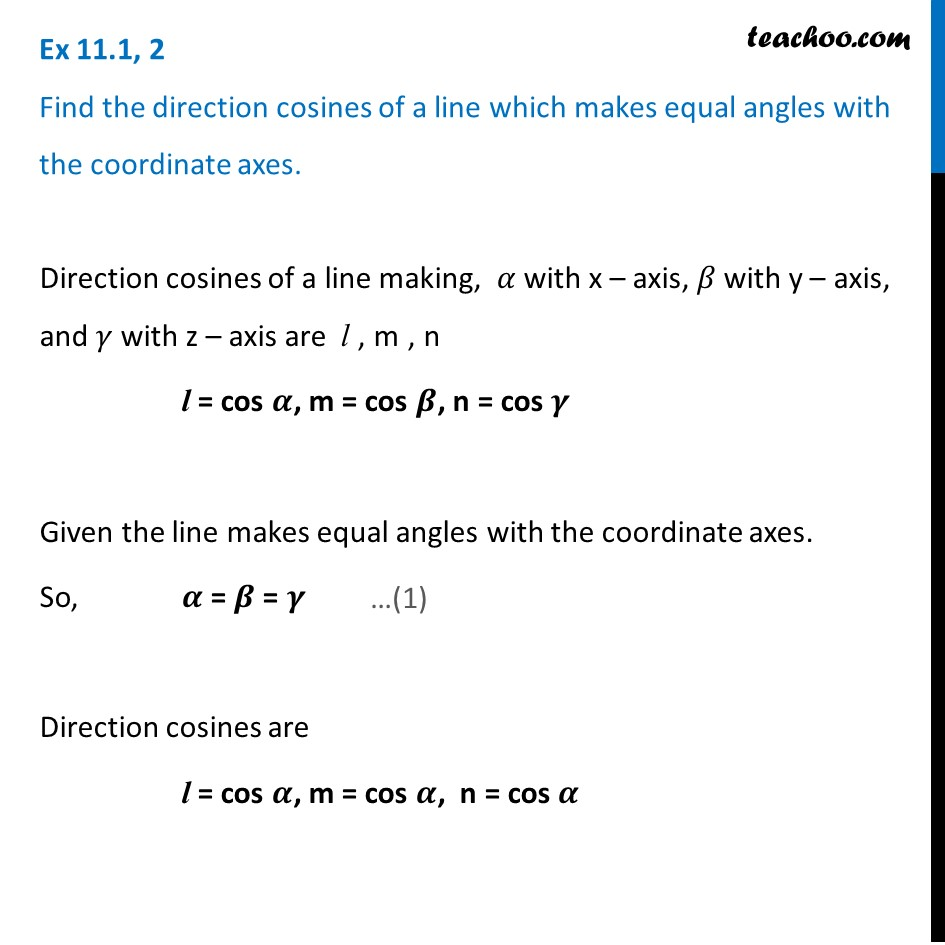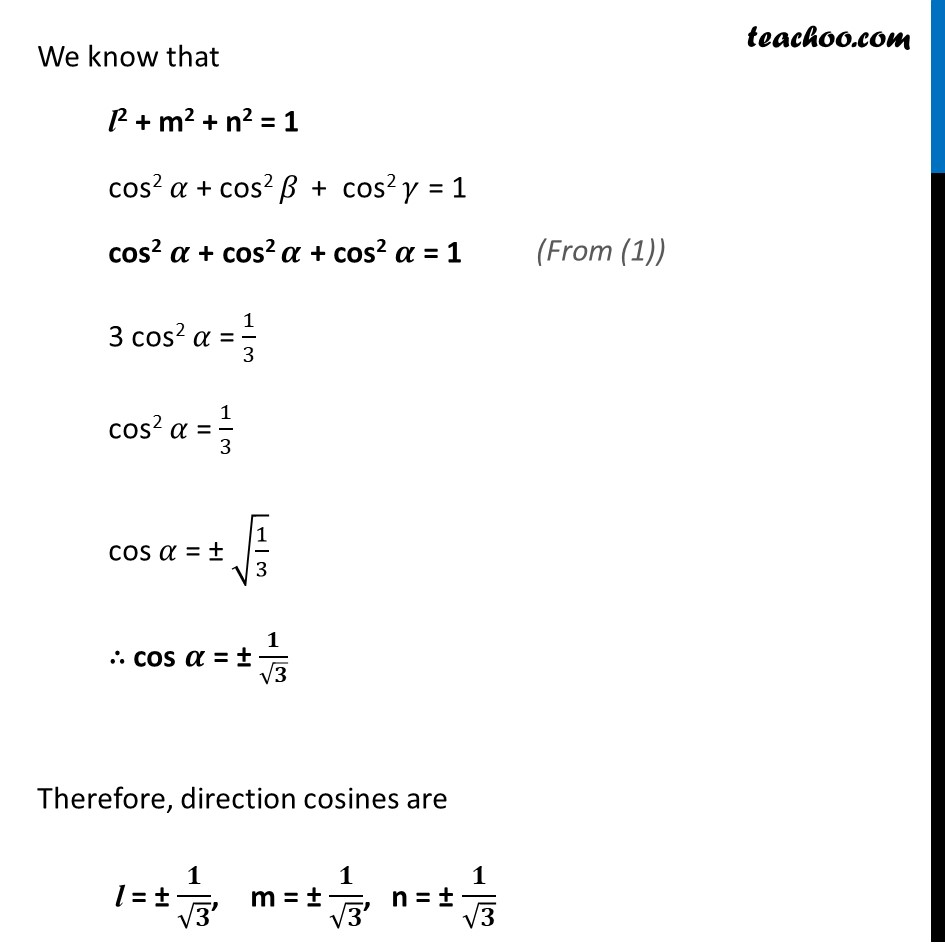Ex 11.1

Chapter 11 Class 12 Three Dimensional Geometry
Serial order wiseLearn in your speed, with individual attention - Teachoo Maths 1-on-1 Class

### Transcript

Ex 11.1, 2 Find the direction cosines of a line which makes equal angles with the coordinate axes.Direction cosines of a line making, 𝛼 with x – axis, 𝛽 with y – axis, and 𝛾 with z – axis are l,m,n l = cos 𝜶, m = cos 𝜷, n = cos 𝜸 Given the line makes equal angles with the coordinate axes. So, 𝜶 = 𝜷 = 𝜸 Direction cosines are l = cos 𝜶, m = cos 𝜶, n = cos 𝜶 We know that l2 + m2 + n2 = 1 cos2 𝛼 + cos2 𝛽 + cos2 𝛾 = 1 cos2 𝜶 + cos2 𝜶 + cos2 𝜶 = 1 3 cos2 𝛼 = 1/3 cos2 𝛼 = 1/3 cos 𝛼 = ± √(1/3) ∴ cos 𝜶 = ± 𝟏/√𝟑 Therefore, direction cosines are l = ± 𝟏/√𝟑, m = ± 𝟏/√𝟑, n = ± 𝟏/√𝟑